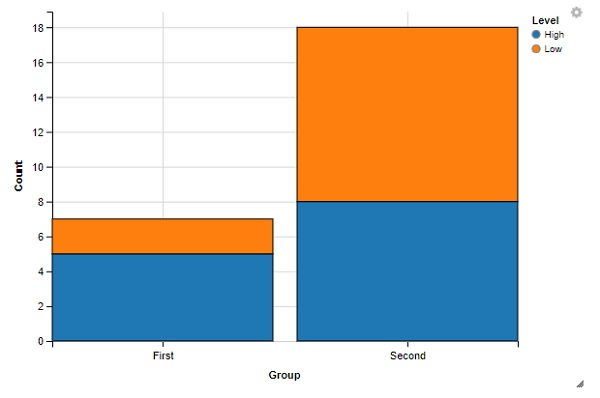# How to create stacked bar chart using ggvis in R?

To create stacked bar chart using ggvis, we can follow the below steps −

• First of all, create a data frame.
• Create the stacked bar chart with layer_bars function of ggvis package.

## Create the data frame

Let's create a data frame as shown below −

Live Demo

Group<-c("First","Second","First","Second")
Level<-c("Low","Low","High","High")
Count<-c(2,10,5,8)
df<-data.frame(Group,Level,Count)
df

On executing, the above script generates the below output(this output will vary on your system due to randomization) −

   Group Level Count
1  First  Low    2
2  Second Low   10
3  First  High   5
4  Second High   8

## Create the stacked bar chart with ggvis

Use layer_bars function of ggvis package to create the stacked bar chart for the data given in data frame df −

Group<-c("First","Second","First","Second")
Level<-c("Low","Low","High","High")
Count<-c(2,10,5,8)
df<-data.frame(Group,Level,Count)
library(ggvis)
df %>% ggvis(x=~Group, y=~Count, fill=~Level) %>% group_by(Level) %>%
layer_bars()

### Output